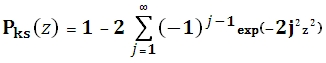# SQL Server Kolmogorov-Smirnov cumulative inverse distribution function

KSPINV

Updated: 29 June 2012

Use KSPINV to calculate the inverse of the Kolmogorov-Smirnov (KS) cumulative distribution function. The equation for the cumulative distribution function is:Syntax
SELECT [wctStatistics].[wct].[KSPINV] (
<@P, float,>)
Arguments
@P
is the value at which to evaluate the function. @P is an expression of type float or of a type that can be implicitly converted to float
Return Types
Float
Remarks
·         @P must greater than zero and less than or equal to one.
·         Pks(0) = 0
·         Pks(8) = 1
Examples
SELECT wct.KSPINV(0.730000328322645) as KSPINV
This produces the following result.
KSPINV
----------------------
1

### SupportCopyright 2008-2023 Westclintech LLC         Privacy Policy        Terms of Service